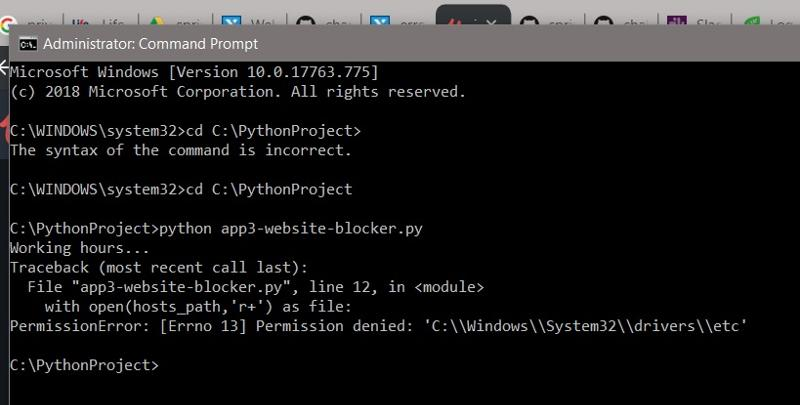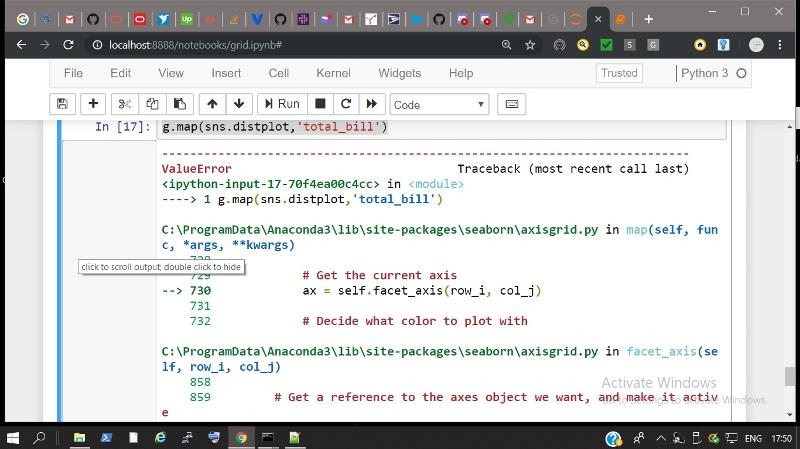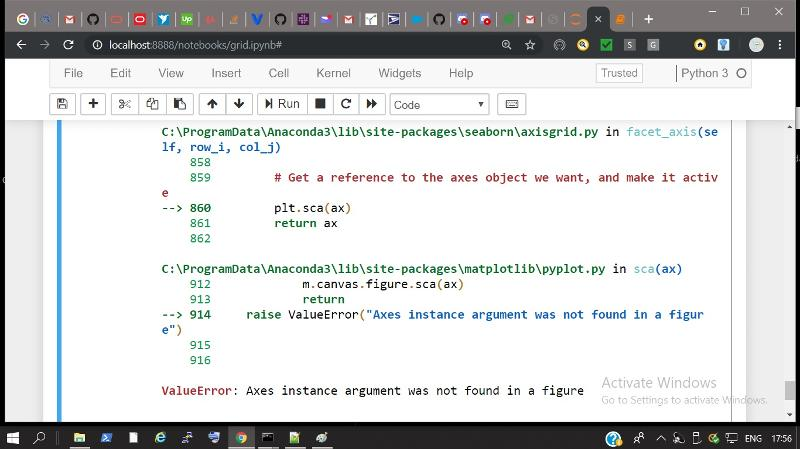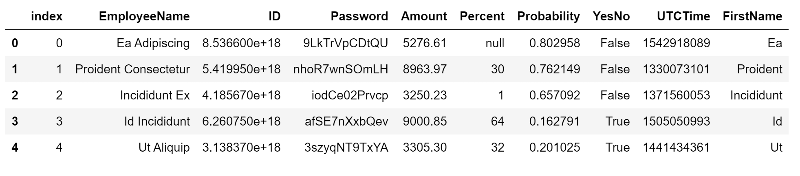Python

4K

Solutions

2K

Contributors

Python is a widely used general-purpose, high-level programming language. Its design philosophy emphasizes code readability, and its syntax allows programmers to express concepts in fewer lines of code than would be possible in other languages. Python supports multiple programming paradigms, including object-oriented, imperative and functional programming or procedural styles. It features a dynamic type system and automatic memory management and has a large and comprehensive set of standard libraries, including NumPy, SciPy, Django, PyQuery, and PyLibrary.

Share tech news, updates, or what's on your mind.
Hey i need to print a sudoku for school but i am having a hard time doing so.

So i made a load function which prints a pre loaded sudoku like this:

['7,9,0,0,0,0,3,0,1',
'0,0,0,0,0,6,9,0,0',
'8,0,0,0,3,0,0,7,6',
'0,0,0,0,0,5,0,0,2',
'0,0,5,4,1,8,7,0,0',
'4,0,0,7,0,0,0,0,0',
'6,1,0,0,9,0,0,0,8',
'0,0,2,3,0,0,0,0,0',
'0,0,9,0,0,0,0,5,4']

For the new function i need to make the function show, which makes the sudoku look like this:

>>> from solver import load, show
7 9 _   _ _ _   3 _ 1
_ _ _   _ _ 6   9 _ _
8 _ _   _ 3 _   _ 7 6

_ _ _   _ _ 5   _ _ 2
_ _ 5   4 1 8   7 _ _
4 _ _   7 _ _   _ _ _

6 1 _   _ 9 _   _ _ 8
_ _ 2   3 _ _   _ _ _
_ _ 9   _ _ _   _ 5 4

This is the code for the load function:

with open(filename) as sudoku_original:

sudoku_original = [line.rstrip('\n') for line in sudoku_original]

sudoku = []
for line in sudoku_original:
sudoku.append(line)
return sudoku

And this is the format of the show function:
def show(sudoku):
# TODO
pass
0
The FOR loop that I have in this Python script to scrape song titles, authors, etc is returning the dictionary with too many records per key. I would like ONE value per key but it is returning all TEN titles, ten authors, ten genres, ten singers from the page. I can see that there are ten DIVs with the class name of col-12, which explains the situation of 10 values within each key, but I cannot figure out a solution to only get one value iterating 10 times in this case. Thank you!

{'title',  'author', 'genre', 'singer'}

Ideally, I want the dictionary like this for example:   {'title':  'I Will Survive', 'author': 'Gloria Gaynor', 'genre': 'dance', 'singer': 'Gloria Gaynor'}

My script:

import scrapy

class hopamspider(scrapy.Spider):
name = 'hopam'
start_urls = ['https://hopamviet.vn/chord/']

def parse(self, response):
all_hopam = response.css('div.col-12')

for song in all_hopam:
title = song.xpath("//h5/a/text()").extract() # main page
author = song.xpath("//h5/small/a/text()").extract() #main page
genre = song.xpath("//span[@class='float-right text-muted small']/text()").extract() #main page
singer = song.xpath("//a[@class='csinger']/text()").extract() #main page
#nextpage = song.xpath("//a[@class='float-right']/@href").extract_first()
#lyrics = …
0
Hey i have a assignment where i have to find a heatwave out of a csv. We can call something a heat wave f there is a period of at least 5 days where the temprature is 25 degrees or more and if in this period there are 3 days with a tempature of 30 degrees or more.
I need to print the first year where a heatwave occured.  Example output: 2019
These are the first lines of the csv:

STAID,SOUID,DATE,TX,Q_TX
162,100522,19010101,-31,0
162,100522,19010102,-13,0
162,100522,19010103,-5,0
162,100522,19010104,-10,0
162,100522,19010105,-18,0
162,100522,19010106,-78,0

DATE is the date in the format year month day
Q is the temprature we are looking for times ten. ( so the temprature is Q/10)

I wrote some code but with this code i cant look if the period has 3 three days with 30 degrees or more. This is the code.

import csv

with open("climate.csv", "r" ) as csvFile:
heat_wave = []
heat_date = []
heat_counter = 0
for row in reader:
row_value = int(row['TX']) / 10
if row_value < 25 and heat_counter > 5:
heat_wave.append()
heat_date.append()
if row_value > 24:
heat_counter += 1
else:
heat_counter = 0

Can anyone help me with this?
0
hi am having the admin running this scriptthis is my script

import time
from datetime import datetime as dt

hosts_temp=r"D:\Dropbox\pp\block_websites\Demo\hosts"
hosts_path="C:\Windows\System32\drivers\etc"
redirect="127.0.0.1"

while True:
if dt(dt.now().year,dt.now().month,dt.now().day,8) < dt.now() < dt(dt.now().year,dt.now().month,dt.now().day,16):
print("Working hours...")
with open(hosts_path,'r+') as file:
for website in website_list:
if website in content:
pass
else:
file.write(redirect+" "+ website+"\n")
else:
with open(hosts_path,'r+') as file:
file.seek(0)
for line in content:
if not any(website in line for website in website_list):
file.write(line)
file.truncate()
print("Fun hours...")
time.sleep(5)
0
Hee i have this csv which takes dates as 20150731 (meaning 31 july 2015).
I want to print these numbers as 7 aug 1990 so the first three letters of the month.
Does anyone know how to do this?
This is my code so far:

import csv

with open("climate.csv", "r" ) as csvFile:
max_temp = 0
min_temp = 0
for row in reader:
row_value = int(row['TX'])
if row_value > max_temp:
max_temp = row_value
max_date = int(row['DATE'])
if row_value < min_temp:
min_temp = row_value
min_date = int(row['DATE'])

And these are the first lines of the csv:

STAID,SOUID,DATE,TX,Q_TX
162,100522,19010101,-31,0
162,100522,19010102,-13,0
162,100522,19010103,-5,0
162,100522,19010104,-10,0
0
I want to get the maximum of a csv but i get the following error:

keyerror: 'TX'

This is my code:
import csv

with open("climate.data", "r" ) as csvFile:
max_temp = 0
min_temp = 0
for row in reader:
row_value = int(row["TX"])
if row_value > max_temp:
max_temp = row_value
if row_value < min_temp:
min_temp = row_value

print (min_temp)

And these are the first lines of the csv:

STAID,SOUID,DATE,TX,Q_TX
162,100522,19010101,-31,0
162,100522,19010102,-13,0
162,100522,19010103,-5,0

Does anyone have a fix for me?
0
I wanna know the longest freezing period from a csv.

The format of the csv is this:

01-06 STAID: Station identifier
08-13 SOUID: Source identifier
15-22 DATE : Date YYYYMMDD
24-28 TX   : Maximum temperature in 0.1 &#176;C
30-34 Q_TX : quality code for TX (0='valid'; 1='suspect'; 9='missing')

STAID,SOUID,DATE,TX,Q_TX
162,100522,19010101,-31,0
162,100522,19010102,-13,0
162,100522,19010103,-5,0
162,100522,19010104,-10,0
162,100522,19010105,-18,0
162,100522,19010106,-78,0

Does anyone know a way to find the longest freezing period and print it in the format:

The longest freezingperiod was 12 days and ended on 29 jun 1999. (example)

Any help would be very much appreciated :)
0
hi am geting this error
df1.plot.line(x=df1.index,y='B',fisize=(12,3))
---------------------------------------------------------------------------
KeyError                                  Traceback (most recent call last)
<ipython-input-13-eb1aa3f6e42a> in <module>
----> 1 df1.plot.line(x=df1.index,y='B',fisize=(12,3))

C:\ProgramData\Anaconda3\lib\site-packages\pandas\plotting\_core.py in line(self, x, y, **kwds)
3003             >>> lines = df.plot.line(x='pig', y='horse')
3004         """
-> 3005         return self(kind='line', x=x, y=y, **kwds)
3006
3007     def bar(self, x=None, y=None, **kwds):

C:\ProgramData\Anaconda3\lib\site-packages\pandas\plotting\_core.py in __call__(self, x, y, kind, ax, subplots, sharex, sharey, layout, figsize, use_index, title, grid, legend, style, logx, logy, loglog, xticks, yticks, xlim, ylim, rot, fontsize, colormap, table, yerr, xerr, secondary_y, sort_columns, **kwds)
2939                           fontsize=fontsize, colormap=colormap, table=table,
2940                           yerr=yerr, xerr=xerr, secondary_y=secondary_y,
-> 2941                           sort_columns=sort_columns, **kwds)
2942     __call__.__doc__ = plot_frame.__doc__
2943

C:\ProgramData\Anaconda3\lib\site-packages\pandas\plotting\_core.py in plot_frame(data, x, y, kind, ax, subplots, sharex, sharey, layout, figsize, use_index, title, grid, legend, style, logx, logy, loglog, xticks, yticks, xlim, ylim, rot, fontsize, …
0
I would like to get the minimum and maximum from a csv using python. Does anyone know how to do this.

The csv is made like this:

01-06 STAID: Station identifier
08-13 SOUID: Source identifier
15-22 DATE : Date YYYYMMDD
24-28 TX   : Maximum temperature in 0.1 &#176;C
30-34 Q_TX : quality code for TX (0='valid'; 1='suspect'; 9='missing')

STAID,    SOUID,    DATE,   TX, Q_TX
162,100522,19010101,  -31,    0

I want the output to be like this:

The max temp was 34.5 degrees on 13 may 1967
So the 05 in the csv must become the first three letters of the month like may.
If anyone could help me with this i would appreciate it alot :)

My code so far:

import csv
from itertools import islice

with open("climate.data", "r") as dataFile, open("climate.csv", "w") as csvFile:
for row in islice(climate, 20, None):
print(row)

0
hi am having error in my script
python mapView.py
Traceback (most recent call last):
File "mapView.py", line 44, in <module>
style_function=lambda x: {'fillColor':'yellow'}))
File "C:\ProgramData\Anaconda3\lib\site-packages\folium\features.py", line 450, in __init__
self.data = self.process_data(data)
File "C:\ProgramData\Anaconda3\lib\site-packages\folium\features.py", line 494, in process_data
': {!r}'.format(data))
ValueError: Cannot render objects with any missing geometries: <_io.TextIOWrapper name='world.json' mode='r' encoding='utf-8-sig'>
this my script
import folium
import pandas

lat = list(data["LAT"])
lon = list(data["LON"])
elev = list(data["ELEV"])

def color_producer(elevetion):
if elevetion < 1000:
return  'green'
elif 1000 <= elevetion < 3000:
return 'orange'
else:
return 'red'

#map = folium.Map(location=[lat, lon], zoom_start=6, tiles="Stamen Terrain")
#map = folium.Map(location=[lat, lon], zoom_start=5, tiles="Stamen Terrain")

map = folium.Map(location=[38.58,-99.09], zoom_start=5, tiles="Mapbox Bright")

#map = folium.Map(location=[0, 0], zoom_start=6)

fg = folium.FeatureGroup(name="My Map")

#for lt,ln,el in zip(lat,lon,elev):
#    fg.add_child(folium.Marker(location=[lt, ln],popup=str(el)+" m",icon=folium.Icon(color=color_producer(el)))

for lt,ln,el in zip(lat,lon,elev):

0
I want to show the 5 most used words in the set listpositives. Can anyone help me with this?

Example output: amazing 20.

def positive_word(tweets, positives):

#prints the top 5 most used positive words

listpositives= []
wordfreq= []

for i in range(len(tweets)):
for j in range(len(tweets[i])):
if tweets[i][j] in positives:
listpositives.append(tweets[i][j])
0
Hello,

Attached is the Python code.  In the attachment:

Number 1 indicates that data was extracted giving appropriate command, and the displayed correctly.
A indicates the command written, and similar commands are given below as well.
Numbers 2 and 3 indicate response from system.

What is this response from system, (sqlite3.Cursor object at 0x03FC10A0), and what is missed over here? What should be expected as a result? There are similar commands below also.

Thanks,
San.
0
hi am having the following error
python mapView.py

File "mapView.py", line 33

^

SyntaxError: invalid syntax

code is
import folium
import pandas

lat = list(data["LAT"])
lon = list(data["LON"])
elev = list(data["ELEV"])

def color_producer(elevetion):
if elevetion < 1000:
return  'green'
elif 1000 <= elevetion < 3000:
return 'orange'
else:
return 'red'

#map = folium.Map(location=[lat, lon], zoom_start=6, tiles="Stamen Terrain")
map = folium.Map(location=[lat, lon], zoom_start=5, tiles="Stamen Terrain")
#map = folium.Map(location=[0, 0], zoom_start=6)

fg = folium.FeatureGroup(name="My Map")

for lt,ln,el in zip(lat,lon,elev):

map.save("map092.html")
0
I am new to Python and I found this program online. So I am trying to understand how hangman dashes gets updated from dashes to letters. I am also trying to understand how the program knows which dashes gets updated to letters.

print("Welcome to Python Hangman")
print()

import random # Needed to make a random choice
from turtle import * #Needed to draw line

WORDS= ("variable", "python", "turtle", "string", "loop")

word = random.choice(WORDS)#chooses randomly from the choice of words
print  ("The word is", len(word), "letters long.")# used to show how many letters are in the random word

ln = len(word)
guessed = dict.fromkeys(word, 0)
print("_ "*ln)
correct = 0
for i in range(1, 9):#gives the amount of guesses allocated
letter = input("Guess a letter ")

if letter in word:
print ("Correct! {} is in the word".format(letter))#if guesses letter is correct print correct
guessed[letter] = 1
correct += 1
if correct == ln:#??
print("Congratulations! you win.\n The word was {}".format(word))
break
else:
print ("Incorrect! {} is not in the word".format(letter))
#if its wrong print incorecct
print(" ".join([ch if guessed[ch] else "_" for ch in word]))
else:
print("You lose!\nThe word was {}".format(word))
0
Hi Experts,

Is there a way to find the highest number in a list then the second highest then the third highest in a list without using sort

Thank you.
0
I am trying to create a csv using python pandas... example pd.to_csv("somefile.csv") to a network shared drive in a windows environment.  The problem is that when I open the windows explorer folder, I don't see the file, but if I use the command prompt, the file is listed.
0
Does Python Programmer requies only Python Knowledge.

I have heard a lot about the importance of learning Python. So I checked the area of Web Development using Python, however I noticed they mostly use Django and some use Flask. It sounds like to me  that Python is just like an Envelope , what is inside is not Python, it is DJango, Flask and probably other Frameworks.

I am Planning to learn Python for Web Development and Network programming ( as I have Network Background) , I believe in Network Programming you can use Python alone, no need for other Frameworks, but in Web Development someone needs to learn other Frameworks especially Django. Hopefullly Python+Django can make me Web Developer too ...

Any opinions, suggestions, orientations about  this topic are very welcome

Thank you
0
This is a learning exercise for me. It has no practical value other than for learning about regex expressions.
Here is a program with several regex patterns.
import re

a = r"stuff<offset>1234</offset><length>78</length>stuff <offset>1000134</offset><length>5678</length>stuff...<offset>11234</offset><length>5678</length>stuff"
r  = re.compile(r"<offset>([^<]+)</offset><length>([^<]+)</length>")
matches = r.findall(a)   #instantiate our matches variable
print(matches)
print(max(r.findall(a), key = lambda x: int(x)))
print("***** done with original working example using <>")
print("")

b = r"stuff[offset]1234[/offset](length)78(/length)stuff [offset]1000134[/offset](length)5678(/length)stuff...[offset]11234[/offset](length)5678(/length)stuff"
rb0 = re.compile(r"[offset]([^\[]+)[/offset][length]([^\[]+)[/length]")
rb1 = re.compile(r"\[offset\]([^\[]+)\[/offset\]\[length\]([^\[]+)\[/length\]")
rb2 = re.compile(r"\[offset\]([^[]+)\[\/offset\]\[length\]([^[]+)\[\/length\]")
rb3 = re.compile(r"\[offset\](\d+)\[\/offset\]\(length\)(\d+)\(\/length\)")

bmatches0 = rb0.findall(b)
bmatches1 = rb1.findall(b)
bmatches2 = rb2.findall(b)
bmatches3 = rb3.findall(b)

print("bmatches0: ",bmatches0)
print("bmatches1: ",bmatches1)
print("bmatches2: ",bmatches2)
print("bmatches3: ",bmatches3)

print(max(rb0.findall(b), key = lambda x: ()))

print(max(rb3.findall(b), key = lambda x: int(x)))
Here is the output. Output: Lines 5,6,7,9 are wrong. Can you please explain why the respective pattern produced the results?
[('1234', '78'), ('1000134', '5678'), ('11234', '5678')]
('1000134', '5678')
***** done with original working example using <>

bmatches0:  [('ffset](length)78(/leng', ')s'), ('ffset](length)5678(/leng', ')s'), ('ffset](length)5678(/leng', ')s')]
bmatches1:  []
bmatches2:  []
bmatches3:  [('1234', '78'), ('1000134', '5678'), ('11234', '5678')]
('ffset](length)78(/leng', ')s')
('1000134', '5678')
Thanks for the help.
Paul
0
I have a text string like this:
"stuff<offset>1234</offset><length>78</length>stuff <offset>1000134</offset><length>5678</length>stuff...<offset>11234</offset><length>5678</length>stuff"
My goal is to find the largest offset value and the corresponding length value.

I know I can write a loop searching for each "<offset>" and extract the value. I was wondering if in python3.7, there is a non-loop approach. (I can  use existing xml parsing code, but this seems simple enough to just use the text string.)

Thanks,
Paul
0
I'm having serious problems to make my kiosk printer (Nippon Primex NP-VK30) work correctly under Ubuntu 19.04.
The manufacturer provides no support for Ubuntu, but I've found on Github a very simple library made in Python.
I attached the Python files to this topic.

I tried to launch "test.py" and my printer worked fine!

Since I need to print some text strings from my C/C++ application, I would like to translate the python code into C/C++ code so I can easily import it in my original application.

Unfortunately, I'm just a beginner and I never coded in Python.
Can you help me, please?

Thank you!

This is the code for test.py:

#!/usr/bin/python
from w2k203dpi import Printer

p = Printer()

p.println('test')

p.bold(True)
p.println('test')
p.bold(False)

p.underline(True)
p.println('test')
p.underline(False)

p.qrcode('test')

p.fullcut()

This is the code for the class file:

class Printer:

def __init__(self):
DEVPATH = '/dev/usb/lp0'
self.f = open(DEVPATH, 'w')
self.mode = 0x00

def raw(self, data):
for i in data:
self.f.write(i)
self.f.flush()

def esc(self, data):
self.raw('\x1b' + data)

def font(self, value):
if value:
self.mode |= (1 << 0)
else:
self.mode &= ~(1 << 0)
self.esc('!' + chr(self.mode))

def bold(self, value):
if value:
self.mode |= (1 << 3)
else:

0
hi am geting the following error
python mapView.py
Traceback (most recent call last):
File "C:\ProgramData\Anaconda3\lib\site-packages\folium\utilities.py", line 59, in validate_location
float(coord)
TypeError: float() argument must be a string or a number, not 'list'

During handling of the above exception, another exception occurred:

Traceback (most recent call last):
File "mapView.py", line 13, in <module>
map = folium.Map(location=[lon, lat], zoom_start=6, tiles="Stamen Terrain")
File "C:\ProgramData\Anaconda3\lib\site-packages\folium\folium.py", line 249, in __init__
self.location = validate_location(location)
File "C:\ProgramData\Anaconda3\lib\site-packages\folium\utilities.py", line 63, in validate_location
.format(coord, type(coord)))
ValueError: Location should consist of two numerical values, but [-121.810997, -121.1110001, -121.7509995, -122.1809998, -121.4909973, -122.0810013, -121.8209991, -121.6910019, -121.8010025, -121.7710037, -121.9309998, -121.8310013, -121.8410034, -121.7710037, -121.7710037, -121.6809998, -121.2210007, -121.8209991, -120.8610001, -122.10099790000001, -120.7509995, -120.6610031, -122.1210022, -110.6709976, -118.7509995, -117.4710007, -117.8010025, -113.5009995, -112.45099640000001, -114.35099790000001, -117.5810013, -121.5709991, -113.2210007, -122.20099640000001, -121.4410019, -121.3610001, -121.60099790000001, -121.5510025, -120.8310013, -121.5009995, -122.7710037, -119.7210007,
0hi am geting this error
g.map(sns.distplot,'total_bill')
ValueError                                Traceback (most recent call last)
<ipython-input-17-70f4ea00c4cc> in <module>
----> 1 g.map(sns.distplot,'total_bill')

C:\ProgramData\Anaconda3\lib\site-packages\seaborn\axisgrid.py in map(self, func, *args, **kwargs)
728
729             # Get the current axis
--> 730             ax = self.facet_axis(row_i, col_j)
731
732             # Decide what color to plot with

C:\ProgramData\Anaconda3\lib\site-packages\seaborn\axisgrid.py in facet_axis(self, row_i, col_j)
858
859         # Get a reference to the axes object we want, and make it active
--> 860         plt.sca(ax)
861         return ax
862

C:\ProgramData\Anaconda3\lib\site-packages\matplotlib\pyplot.py in sca(ax)
912             m.canvas.figure.sca(ax)
913             return
--> 914     raise ValueError("Axes instance argument was not found in a figure")
915
916

ValueError: Axes instance argument was not found in a figure0
We receive email which has ".eml" files I need to open "eml" files and extract all the "pdf" attachments.  (i have email folder as "attachments" where i have emails"; I am using office 2016

I am currently saving all the emails to a folder by using macro "https://gallery.technet.microsoft.com/office/Save-attachments-from-5b6bf54b"however I need to extract all pdf files from eml files which are inside ".msg" file

tried this did not work: https://stackoverflow.com/questions/19255083/vba-outlook-extracting-attachments-from-eml-fileshttps://gist.githubusercontent.com/urschrei/5258588/raw/aba67931890a91692e21e9edf45c09d9d1f145ca/parseml.py
0
Experts,

Can you assist me in creating a function 'employeefirstName (df) in Python/Pandas that will extract the first name out of the EmployeeName field from the attached dataset that will

1.      Extracts the firstname, in all lowercase, from the EmployeeName column in the df dataset.
a.      The EmployeeName consists of letters, a space and then more letters.(e.g  Peter Jones)
b.      The letters before the space is assumed to be first name (e.g Peter in the example above)
2.      Returns the results with the following schema
Column              Type
FirstName      string

The output should look something like
FirstName
sarah
peter
jane
roger

I have attempted to achieve the above with the following code:

import numpy as np
import pandas as pd

def employeeFirstName(df):
df['FirstName'] = 0
for i in range(len(df['EmployeeName'])):
df['FirstName'][i] = df['EmployeeName'][i].split(' ')
return df

newData = employeeFirstName(df)

However, I keep on getting the results shown in the imagecc3_stringfunctions.csv
0
In fact this are really three question:
1.) is grep -f memory friendly? (so could I avoid writing any python code)
2.) Does somebody have already a working solution for below problem?
3.) Could you make suggestions or review my code as soon as it starts working.

I'm trying to find a solution, that works for really huge files. (will not  use more RAM if files have more lines)
The problem: identify all lines, that exist in the first file, but don't exist
in the second one. In none of the files the same line can occur twice

Here a small example:

file1 (f1.txt)
aba
abb
abd
abf
one
two
three
four
five
six
seven

File2 (f2.txt)
abd
abe
eleven
four
eight
one
six

The expected result (order is not relevant)
aba
abb
abf
five
seven
three
two

I have already three approaches and an idea for the fourth one
1.) use grep -v -f f2.txt  f1.txt but I know nothing at all about memory consumption
2.) both files can be read into memory (so not suitable for huge files)
3.) the first file is huge the file fits into memory (so not suitable if the second file is huge)
4.) I'm working on it. In the moment the solution does not work as I didn't finish it. The idea es explained below

I have an idea of how to implement a solution, but wanted to know whether anybody has already written such code or knows of a standard solution.

My idea is to sort both files (the default linux sort is quite smart and ensures, that it works …
0

Python

4K

Solutions

2K

Contributors

Python is a widely used general-purpose, high-level programming language. Its design philosophy emphasizes code readability, and its syntax allows programmers to express concepts in fewer lines of code than would be possible in other languages. Python supports multiple programming paradigms, including object-oriented, imperative and functional programming or procedural styles. It features a dynamic type system and automatic memory management and has a large and comprehensive set of standard libraries, including NumPy, SciPy, Django, PyQuery, and PyLibrary.

Top Experts In
Python
Monthly